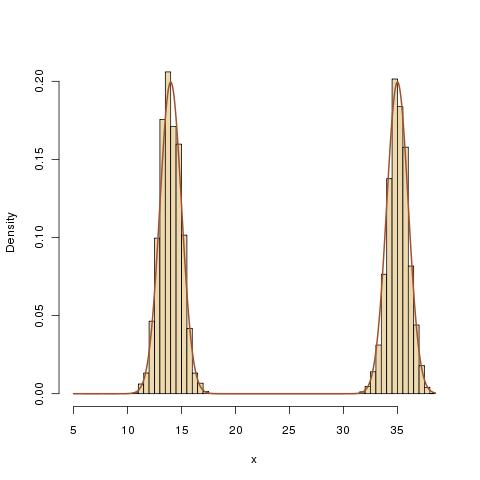Want to share your content on R-bloggers? click here if you have a blog, or here if you don't.A new Metropolis-Hastings algorithm that I would call “universal” was posted by Somak Dutta yesterday on arXiv. Multiplicative random walk Metropolis-Hastings on the real line contains a different Metropolis-Hastings algorithm called the random dive. The proposed new value x’ given the current value x is defined by$begin{cases} xepsilon &text{with probability } 1/2\ x/epsilon &text{with probability } 1/2end{cases}$

when$epsilon$ is a random variable on$(-1,1)$. Thus, at each iteration, the current value is either shrunk or expanded by a random amount. When I read the paper in the Paris metro, while appreciating that this algorithm could be geometrically ergodic as opposed to the classical random walk algorithm, I was not convinced by the practical impact of the method, as I thought that the special role of zero in the proposal was not always reflected in the target. Especially when considering that the proposal is parameter-free. However, after running the following R program on a target centred away from zero, I found the proposal quite efficient.

f=function(x){.5*dnorm(x,mean=14)+.5*dnorm(x,mean=35)}
Nsim=10^5
x=rep(5,Nsim)
for (t in 2:Nsim){
coef=runif(1,min=-1)^sample(c(-1,1),1)
prop=x[t-1]*coef
prob=abs(coef)*f(prop)/f(x[t-1])
x[t]=x[t-1]
if (runif(1) }
hist(x,pro=T,nclass=113,col=”wheat2″)

Obviously, it is difficult to believe that this extension will keep working similarly well when the dimension increases but this is an interesting way of creating a heavy tail proposal.

Filed under: R, Statistics Tagged: geometric ergodicity, Metropolis-Hastings, R, random walk, randomness